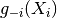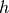# statsmodels.nonparametric.kernel_regression.KernelReg.cv_loo¶

`KernelReg.``cv_loo`(bw, func)[source]

The cross-validation function with leave-one-out estimator.

Parameters: bw: array_like Vector of bandwidth values. func: callable function Returns the estimator of g(x). Can be either `_est_loc_constant` (local constant) or `_est_loc_linear` (local_linear). L: float The value of the CV function.

Notes

Calculates the cross-validation least-squares function. This function is minimized by compute_bw to calculate the optimal value of bw.

For details see p.35 in 

..math:: CV(h)=n^{-1}sum_{i=1}^{n}(Y_{i}-g_{-i}(X_{i}))^{2}

whereis the leave-one-out estimator of g(X) andis the vector of bandwidths